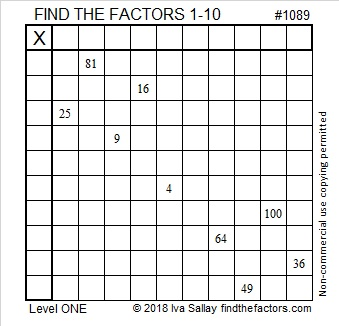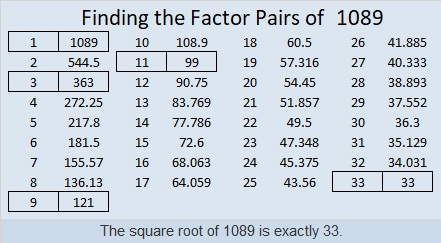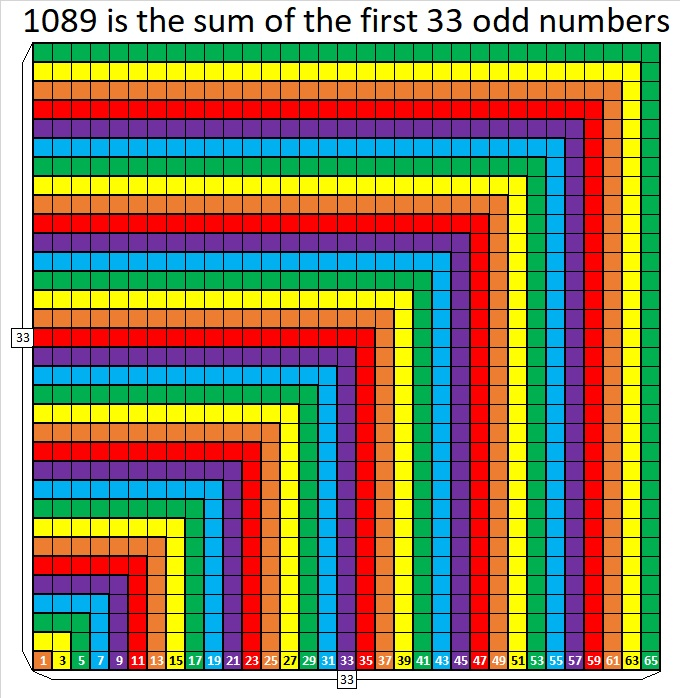# 1089 Perfect Squares

The nine clues in today’s puzzle are all perfect squares. They are all you need to find all the factors that can turn this puzzle into a multiplication table . . . but with the rows and columns not in the typical order:Print the puzzles or type the solution in this excel file: 10-factors-1087-1094

1089 is also a perfect square.

The previous perfect square was 32² = (33 – 1)² = 33² + 1 – 2(33) = 1024
The next perfect square will be 34² = (33 + 1)² = 33² + 1 + 2(33) = 1156

Here’s a little more about the number 1089:

• 1089 is a composite number.
• Prime factorization: 1089 = 3 × 3 × 11 × 11, which can be written 1089 = 3²× 11²
• The exponents in the prime factorization are 2 and 2. Adding one to each and multiplying we get (2 + 1)(2 + 1) = 3 × 3 = 9. Therefore 1089 has exactly 9 factors.
• Factors of 1089: 1, 3, 9, 11, 33, 99, 121, 363, 1089
• Factor pairs: 1089 = 1 × 1089, 3 × 363, 9 × 121, 11 × 99, or 33 × 33
• 1089 is a perfect square. √1089 = 331, 9, 121, and 1089 are all perfect square factors of 1089.

1089 can be 3 × 3 perfect squares arranged on an 11 × 11 perfect square grid:1089 can also be 11 × 11 perfect squares arranged on a 3 × 3 perfect square grid:Not only is 1089 the 33rd perfect square, but it is also the sum of the first 33 odd numbers. Note that the nth perfect square is also the sum of the first odd numbers:I’m not attempting to make a picture of this nine-sided shape, but 1089 is the 18th nonagonal number because 18(7(18) – 5)/ 2 = 1089,
or written another way 7(18²)/2 – 5(18)/2 = 1089.

1089 is the sum of five consecutive prime numbers:
199 + 211 + 223 + 227 + 229 = 1089

OEIS.org informs us that 9 × 1089 = 9801

1089 looks rather square when it is written in several other bases:
It’s 900 in BASE 11 because 9(11²) = 1089,
441 in BASE 16 because  4(16²) + 4(16) + 1(1) = 1089,
169 in BASE 30 because 1(30²) + 6(30) + 9(1) = 1089,
144 in BASE 31 because 1(31²) + 4(31) + 4(1) = 1089,
121 in BASE 32 because 1(32²) + 2(32) + 1(1) = 1089,
100 in BASE 33 because 1(33²) = 1089

This site uses Akismet to reduce spam. Learn how your comment data is processed.